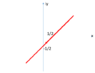# Problem with Complex contour integration

• Joker93

## Homework Statement

I want to compute ##I=\int_C \dfrac{e^{i \pi z^2}}{sin(\pi z)}##, where C is the path in the attached figure (See below). I want to compute this by converting the integral to one whose integration variable is real.

## Homework Equations

There are not more relevant equations.

## The Attempt at a Solution

From the path of the integration, we have that ##y=x-\frac{1}{2}##. Thus ##z=x+iy=x(1+i)-\frac{i}{2}##. With this, we can write the integral as
##I=-(1+i)\int_{-\infty}^{+\infty} \dfrac{e^{i \pi (x(1+i)-\frac{i}{2})^2}}{sin(\pi (x(1+i)-\frac{i}{2}))}##.
where we pick up a minus sign due to reversing the direction of integration.
Is this correct? I am using this integral for a more general proof, but it does not seem to fit nicely with the proof. In particular, what makes things ugly is the fact that when we expand the sin function in the denominator of the integrand function, we get things like ##e^{\pm \pi/2}##, which makes me feel not-so-sure about the steps leading from ##z## to ##x##.
Thank you!

#### Attachments

•Untitled.png
1.8 KB · Views: 347

## Homework Statement

I want to compute ##I=\int_C \dfrac{e^{i \pi z^2}}{sin(\pi z)}##, where C is the path in the attached figure (See below). I want to compute this by converting the integral to one whose integration variable is real.

## Homework Equations

There are not more relevant equations.

## The Attempt at a Solution

From the path of the integration, we have that ##y=x-\frac{1}{2}##. Thus ##z=x+iy=x(1+i)-\frac{i}{2}##. With this, we can write the integral as
##I=-(1+i)\int_{-\infty}^{+\infty} \dfrac{e^{i \pi (x(1+i)-\frac{i}{2})^2}}{sin(\pi (x(1+i)-\frac{i}{2}))}##.
where we pick up a minus sign due to reversing the direction of integration.
Is this correct? I am using this integral for a more general proof, but it does not seem to fit nicely with the proof. In particular, what makes things ugly is the fact that when we expand the sin function in the denominator of the integrand function, we get things like ##e^{\pm \pi/2}##, which makes me feel not-so-sure about the steps leading from ##z## to ##x##.
Thank you!

Everything you did is perfectly legitimate.

To go further, you could re-express ##\exp(i \pi (x(1+i) - \frac 1 2)^2) ## by expanding out the exponent in real and imaginary terms. That will turn out to give a nicer-looking result, although it will not make the integral any more "do-able".

As for expansion of the denominator: I did it using the computer algebra package "Maple" and obtained
$$\text{denominator} = \cos(\pi x) \cosh(\pi x) - i \: \sin(\pi x) \sinh(\pi x)$$
There are no "##\pm##" ambiguities when you carry out the simplifications all the way to the bitter end. (Getting the result above by manual computation would not be difficult, but I am tired of doing such stuff by hand---hence the use of a computer algebra package.)

•Joker93